Balbharti Maharashtra State Board 12th Commerce Book Keeping & Accountancy Solutions Chapter 3 Reconstitution of Partnership (Admission of Partner) Textbook Exercise Questions and Answers.

## Maharashtra State Board 12th Book Keeping & Accountancy Solutions Chapter 3 Reconstitution of Partnership (Admission of Partner)

1. Objetive type questions.

A. Select the most appropriate alternatives from the following and rewrite the sentences.

Question 1.
Anuj and Eeshan are two partners sharing profits and losses in the ratio of 3 : 2. They decided to admit Aaroh for 1/5th share, the new profit sharing ratio will be __________
(a) 12 : 8 : 5
(b) 4 : 3 : 1
(c) 12 : 8 : 1
(d) 12 : 3 : 1
(a) 12 : 8 : 5

Question 2.
Excess of proportionate capital over actual capital represents __________
(a) equal capital
(b) surplus capital
(c) deficit capital
(d) gain
(c) deficit capitalQuestion 3.
__________ is credited when unrecorded asset is brought into business.
(a) Revaluation Account
(b) Balance Sheet
(d) Partners Capital Account
(a) Revaluation Account

Question 4.
When goodwill is withdrawn by the partner __________ account is credited.
(a) Revaluation
(b) Cash/Bank
(c) Current
(b) Cash/Bank

Question 5.
If asset is taken over by the partner __________ Account is debited.
(a) Revaluation
(b) Capital
(c) Asset
(d) Balance Sheet
(b) Capital

B. Write the word/phrase/term, which can substitute each of the following statements.

Question 1.
The method under which calculation of goodwill is done on the basis of extra profit earned above the normal profit.
Super Profit Method

Question 2.
An account is opened to adjust the value of assets and liabilities at the time of admission of a partner.
Revaluation A/c or Profit and Loss A/cQuestion 3.
The reputation of a business is measured in terms of money.
Goodwill

Question 4.
The ratio in which general reserve is distributed to the old partners.
Old Ratio

Question 5.
Name the method of the treatment of goodwill where a new partner will bring his share of goodwill in cash.

Question 6.
The proportion in which old partners make a sacrifice.
Sacrifice Ratio

Question 7.
Capital employed × NRR/100 = __________
Normal Profit

Question 8.
An Account is debited when the partner takes over the asset.
Partner’s Capital A/c or Partner’s Current A/cQuestion 9.
Profit and Loss Account balance appearing on the liability side of the Balance Sheet.
Undistributed Profit or Accumulated Profit

Question 10.
Old ratio – New ratio = __________
Sacrifice Ratio

C. State True or False with reasons:

Question 1.
A new partner can bring capital in cash or kind.
This statement is True.
As per the provision of partnership deed, when any person is admitted in the firm, he has to bring some amount as capital which can be in cash or in-kind of assets to get rights in the assets and definite share in the future profit of the firm.

Question 2.
When goodwill is paid privately to the partners, it is not recorded in the books.
This statement is True.
When goodwill is paid privately to the partners, by a newly admitted person, then in such case no transaction takes place in the business, and the firm as such is not all benefited. Hence it is not recorded in the books of accounts.

Question 3.
The gain ratio is calculated at the time of admission of a partner.
This statement is False.
At the time of admission of a person, in the business, sacrifices are made by the old partners in favour of the new partner. It means there is no question of any gain to the partners, so we can say that the Gain ratio is not calculated at the time of admission of a partner.Question 4.
Revaluation profit is distributed among all partners including new partners.
This statement is False.
Revaluation profit arises due to efforts and hardworking of the old partners in the past and hence profit earned on revaluation of assets and liabilities at the time of admission of a person as a partner in the business belongs to old partners. So, such profit is not distributed among all partners including the new partners. It is distributed only among old partners.

Question 5.
Change in the relationship between the partners is called as Reconstitution of Partnership.
This statement is True.
When any person joins the business as a partner, a change in the relationship takes place. The old agreement is terminated and a new agreement is prepared. There is the change in profit or loss sharing ratio and relationship of the partners which is known as Reconstitution of Partnership.

Question 6.
New partners always bring their share of goodwill in cash.
This statement is False.
When a new person is admitted to the partnership firm, the old partners surrender a certain share in profit and give it to a new partner. In exchange for that new partner is required to bring goodwill in cash or in kind. If he is unable to bring cash for goodwill, then Goodwill is raised and adjusted to the new partner’s capital A/c.

Question 7.
When the goodwill is written off, the goodwill account is debited.
This statement is False.
To write off goodwill means to decrease or wipe out the value of goodwill. When goodwill as an asset of the business is raised, Goodwill A/c is debited in the books of Account. Conversely, when Goodwill is written off from the business, the Goodwill A/c is credited in the books of business.

Question 8.
The new ratio minus the old ratio is equal to the sacrifice ratio.
This statement is False.
When a new partner is admitted, old partners have to sacrifice their profit share in favour of the new partner and their old ratio gets reduced and whatever ratio is left becomes a new ratio. Hence, as per equation:
New Ratio = Old Ratio – Sacrifice Ratio.
By interchanging the terms,
Sacrifice Ratio = Old Ratio – New Ratio.Question 9.
Usually, when a new partner is admitted to the firm, there will be an increase in the capital of the firm.
This statement is True.
When a new partner is admitted to the firm, he brings his share of capital and goodwill, in cash or in-kind, to enjoy the right of sharing the future profit, and hence there will be an increase in the capital of the firm.

Question 10.
Cash/Bank Account is credited when goodwill is withdrawn by the old partners.
This statement is True.
When a new partner brings his share of goodwill, old partners have the right to withdraw it in cash. Therefore, when old partners withdraw the amount of goodwill, cash goes out from the firm and not goodwill. Hence Cash/Bank A/c is credited.

D. Find the odd one.

Question 1.
General reserve, Creditors, Machinery, Capital
Machinery

Question 2.
Decrease in Furniture, Patents wrote off, Increase in Bills payable, R.D.D. written off
R.D.D. written offQuestion 3.
Super profit method, Valuation method, Average profit method, Fluctuating capital method
Fluctuating capital method

E. Calculate the following:

Question 1.
A and B are partners in a firm sharing profit and losses in the ratio of 1 : 1. C is admitted. A surrenders 1/4th share and B surrenders 1/5th of his share in favour of C. Calculate new profit sharing ratio.
Solution:
Old ratio of A and B = 1 : 1 or $$\frac{1}{2}$$ : $$\frac{1}{2}$$
A’s sacrifice = $$\frac{1}{4} \times \frac{1}{2}=\frac{1}{8}$$
B’s sacrifice = $$\frac{1}{5} \times \frac{1}{2}=\frac{1}{10}$$
Sacrificing ratio of A and B = $$\frac{1}{8}$$ : $$\frac{1}{10}$$ = 5 : 4
C’s share = A’s share + B’s share = $$\frac{1}{8}+\frac{1}{10}=\frac{5+4}{40}=\frac{9}{40}$$
A’s new share = Old ratio – Sacrifice ratio = $$\frac{1}{2}-\frac{1}{8}=\frac{4-1}{8}=\frac{3}{8}$$
B’s new share = Old ratio – Sacrifice ratio = $$\frac{1}{2}-\frac{1}{10}=\frac{5-1}{10}=\frac{4}{10}$$
Therefore, New ratio of A, B and C = $$\frac{3}{8}: \frac{4}{10}: \frac{9}{40}$$ = 15 : 16 : 9
(Making denominator equal)

Question 2.
Anika and Radhika are partners sharing profit in the ratio of 5 : 1. They decide to admit Sanika to the firm for 1/5th share. Calculate the Sacrifice ratio of Anika and Radhika.
Solution:
Balance = 1 – share of new partner
= 1 – $$\frac{1}{5}$$
= $$\frac{4}{5}$$ (Remaining share)
New ratio = Old ratio x Balance of 1
Anika’s New ratio = $$\frac{5}{6} \times \frac{4}{5}=\frac{20}{30}$$
Radhika’s New ratio = $$\frac{1}{6} \times \frac{4}{5}=\frac{4}{30}$$
Sanika’s New ratio = $$\frac{1}{5} \times \frac{6}{6}=\frac{6}{30}$$ (Making denominator equal)
∴ New Profit and Loss ratio = $$\frac{20}{30}: \frac{4}{30}: \frac{6}{30}$$ = 20 : 4 : 6 i.e. 10 : 2 : 3
Sacrifice ratio = old ratio – New ratio
Anika’s Sacrifice ratio = $$\frac{5}{6}-\frac{20}{30}=\frac{25-20}{30}=\frac{5}{30}$$
Radhika’s Sacrifice ratio = $$\frac{1}{6}-\frac{4}{30}=\frac{5-4}{30}=\frac{1}{30}$$
∴ Sacrifice ratio = $$\frac{5}{30}: \frac{1}{30}$$ = 5 : 1Question 3.
Pramod and Vinod are partners sharing profits and losses in the ratio of 3 : 2. After the admission of Ramesh the New ratio of Pramod, Vinod and Ramesh are 4 : 3 : 2. Find out the Sacrifice ratio.
Solution:
Sacrifice Ratio = Old ratio – New ratio
Pramod’s Sacrifice ratio = $$\frac{3}{5}-\frac{4}{9}=\frac{27-20}{45}=\frac{7}{45}$$
Vinod’s Sacrifice ratio = $$\frac{2}{5}-\frac{3}{9}=\frac{18-15}{45}=\frac{3}{45}$$
∴ Sacrifice ratio = $$\frac{7}{45}: \frac{3}{45}$$ = 7 : 3.

Question 1.
What is Revaluation Account?
An account opened and operated by any partnership firm for recording changes in the value of assets and liabilities and to ascertain profit or loss made on revaluation of assets and liabilities is called Revaluation Account.

Question 2.
What is meant by Reconstitution of Partnership?
Reconstitution of partnership means a change in the relationship between/among partners and in the form of partnership.

Question 3.
Why is the new partner admitted?
A new partner is admitted to the existing partnership firm to increase the capital resources of the firm and to secure advantages of a new entrant’s skill and business connections, i.e. goodwill.

Question 4.
What is the sacrifice ratio?
A ratio that is surrendered or given up by the old partners in favour of a newly admitted partner is called the sacrifice ratio.Question 5.
What do you mean by raising the goodwill at the time of admission of a new partner?
Raising the Goodwill at the time of admission of a new partner means debiting Goodwill Account up to the value it is raised and crediting. Old partners’ Capital Accounts in their old ratio in the books of the firm.

Question 6.
What is the super profit method of calculation of goodwill?
Super profit method of calculation of Goodwill is a method in which Goodwill is valued at a certain number of years purchases of the super profit of the partnership firm.

Question 7.
When is the ratio of sacrifice calculated for the distribution of goodwill?
The ratio of sacrifice is calculated when the benefits of goodwill contributed by a new partner in cash is to be transferred to existing partners’ Capital/Current Account.

Question 8.
What is the treatment of accumulated profits at the time of admission of a partner?
Accumulated profits at the time of admission of a partner are transferred to old partners’ Capital/Current Accounts in their old profit sharing ratio.

Question 9.
State the ratio in which the old partner’s Capital A/c will be credited for goodwill when the new partner does not bring his share of goodwill in cash.
When the new partner does not bring his share of goodwill in cash, Goodwill is raised up to a certain value and credited to old partners’ Capital/Current A/cs in their old profit sharing ratio.Question 10.
What does the excess of debit over credits in the Profit and Loss Adjustment Account indicate?
The excess of debit over credits in the Profit and Loss Adjustment Account indicates loss on revaluation of assets and liabilities.

G. Complete the table.

Question 1.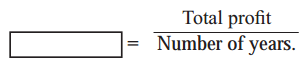Average Profit = $$\frac{Total Profit}{Number of years}$$

Question 2.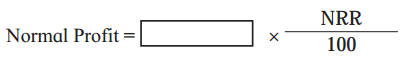Normal Profit = Capital Employed × $$\frac{NPR}{100}$$

Question 3.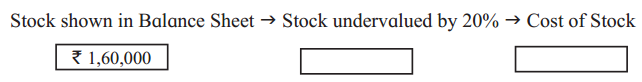The stock shown in Balance Sheet → Stock undervalued by 20% → Cost of Stock
₹ 1,60,000 → ₹ 40,000 → ₹ 2,00,000

Practical Problems

Question 1.
Vikram and Pradnya share profits and losses in the ratio 2 : 3 respectively. Their Balance Sheet as of 31st March 2018 was as under.
Balance Sheet as of 31st March 2018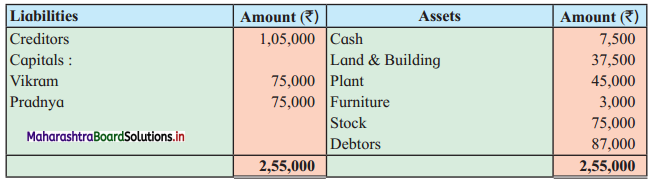They agreed to admit Avani as a partner on 1st April 2018 on the following terms:
1. Avani shall have 1/4th share in future profits.
2. He shall bring ₹ 37,500 as his capital and ₹ 30,000 as his share of goodwill.
3. Land and building to be valued at ₹ 45,000 and furniture to be depreciated by 10%.
4. Provision for bad and doubtful debts is to be maintained at 5% on the Sundry Debtors.
5. Stock to be valued ₹ 82,500.
The Capital A/c of all partners to be adjusted in their new profit and loss ratio and excess amount be transferred to their loan accounts.
Prepare Profit and Loss Adjustment Account, Capital Accounts, and New Balance Sheet.
Solution:
In the books of Partnership Firm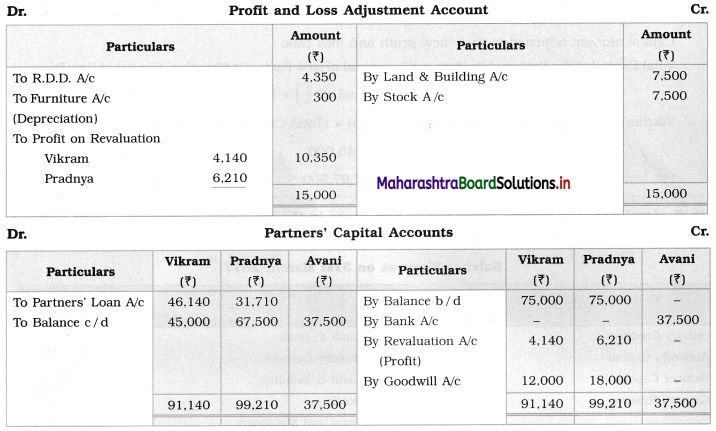Balance Sheet as of 1st April 2018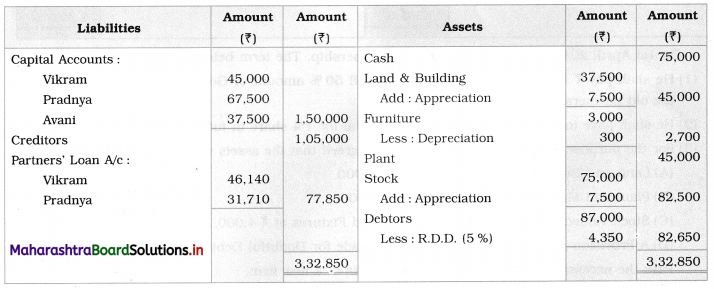Working Notes:
1. Calculation of new profit ratio = 1 – share of new partner
= 1 – $$\frac{1}{4}$$
= $$\frac{3}{4}$$ (Remaining share)
New ratio = old ratio × balance 1 (Remaining share)
Vikram’s new ratio = $$\frac{2}{5} \times \frac{3}{4}=\frac{6}{20}$$
Pradnya’s new ratio = $$\frac{3}{5} \times \frac{3}{4}=\frac{9}{20}$$
Avani’s ratio = $$\frac{1}{4}=\frac{1}{4} \times \frac{5}{5}=\frac{5}{20}$$
∴ New profit sharing ratio = 6 : 9 : 5.
Capital amount adjusted in their new profit and loss ratio:
Total Capital of the Partnership Firm = (Reciprocal of New Partner’s Share) × (Capital of New Partner)
= (Reciprocal of $$\frac{1}{4}$$) × 37,500
= 4 × 37,500
= ₹ 1,50,000
Vikram’s Capital balance = (Vikram’s New Ratio) × (Total Capital of the firm)
= $$\frac{6}{20}$$ × 1,50,000 = ₹ 45,000
Pradnya’s Capital balance = $$\frac{9}{20}$$ × 1,50,000 = ₹ 67,500Question 2.
Amalendu and Sameer share profits and losses in the ratio 3 : 2 respectively. Their Balance Sheet as of 31st March 2017 was as under:
Balance Sheet as of 31st March 2017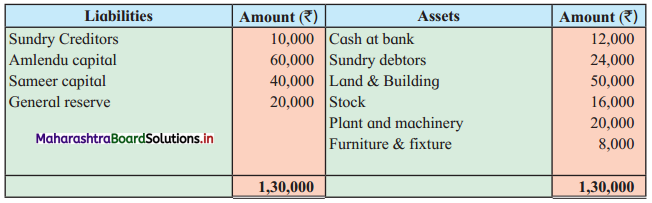On 1st April 2017 they admit Paresh into partnership. The term being that:
1. He shall pay ₹ 16,000 as his share of Goodwill 50% amount of Goodwill shall be withdrawn by the old partners.
2. He shall have to bring in ₹ 20,000 as his Capital for 1/4 share in future profits.
3. For the purpose of Paresh’s admission it was agreed that the assets would be revalued as follows:
A. Land and Building is to be valued at ₹ 60,000.
B. Plant and Machinery to be valued at ₹ 16,000.
C. Stock valued at ₹ 20,000 and Furniture and Fixtures at ₹ 4,000.
D. A Provision of 5% on Debtors would be made for Doubtful Debts.
Pass the necessary Journal Entries in the books of a new firm.
Solution:
Journal entries in the books of Partnership Firm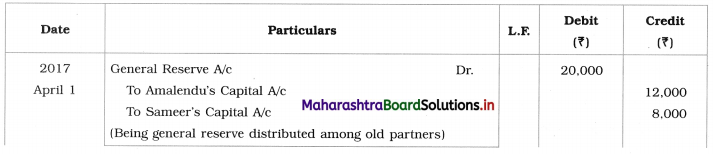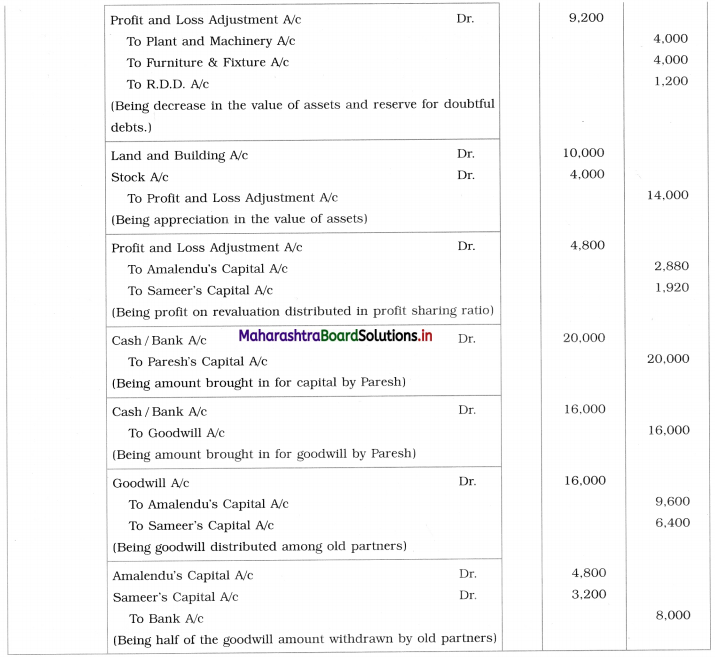Working Notes: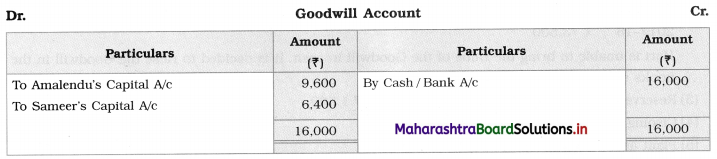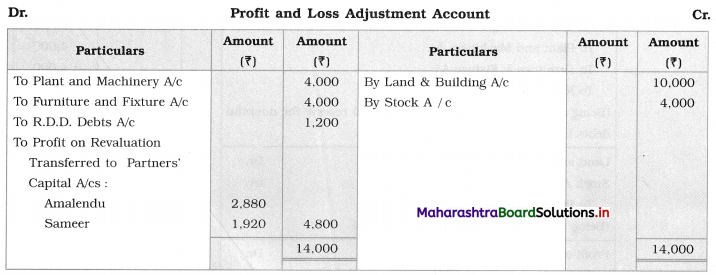Question 3.
Vasu and Viraj share profits and losses in the ratio of 3 : 2 respectively. Their Balance Sheet as on 31st March, 2019 was as under:
Balance Sheet as on 31st March, 2019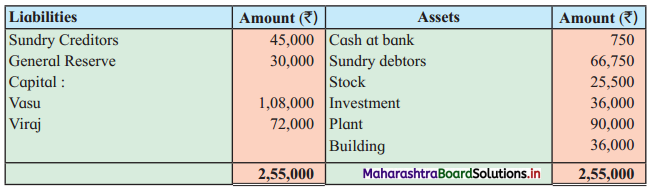They admit Hari into partnership on 1-4-2019. The terms being that:
1. He shall have to bring in ₹ 60,000 as his Capital for 1/4 share in future profits.
2. Value of Goodwill of the firm is to be fixed at the average profits for the last three years.
The Profit were:
2015-16 – ₹ 48,000
2016-17 – ₹ 81,000
2017-18 – ₹ 73,500
Hari is unable to bring the value of Goodwill in cash. It is decided to raise Goodwill in the books of accounts.
3. Reserve for Doubtful debts is to be created at ₹ 1,500.
4. Closing stock is valued at ₹ 22,500.
5. Plant and Building are to be depreciated by 5%.
Prepare Profit and Loss-Adjustment A/c, Capital Accounts of Partners and Balance Sheet of the new firm.
Solution:
In the books of the firm __________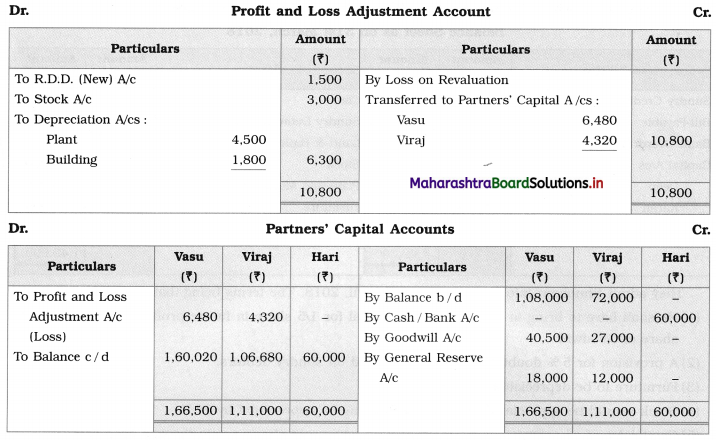Balance Sheet as on 1st April 2019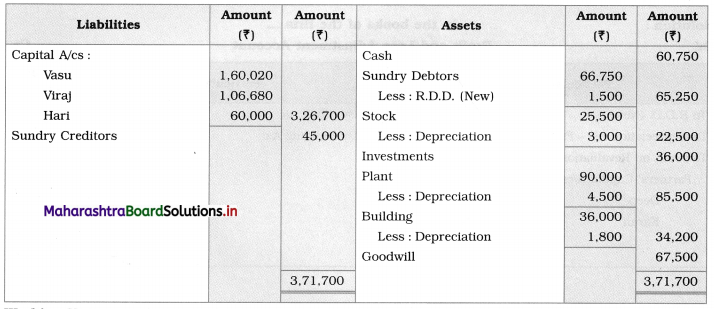Working Notes:
1. Average Profit = $$\frac{Total Profit}{No. of years}$$
= $$\frac{48,000+81,000+73,500}{3}$$
= ₹ 67,500
∴ Goodwill value = ₹ 67,500
Vasu’s share in Goodwill = ₹ 40,500 (67,500 × $$\frac{3}{5}$$)
Viraj’s share in Goodwill = ₹ 27,000 (67,500 × $$\frac{2}{5}$$)

2. Hari is not able to bring a share in goodwill and it is decided to raise the goodwill in the book.
Therefore, Goodwill is recorded in the Asset side ₹ 67,500.Question 4.
Mr. Deep & Mr. Karan were in partnership sharing profits & losses in the proportion of 3 : 1 respectively. Their Balance Sheet on 31st March 2018 stood as follows:
Balance Sheet as of 31st March 2018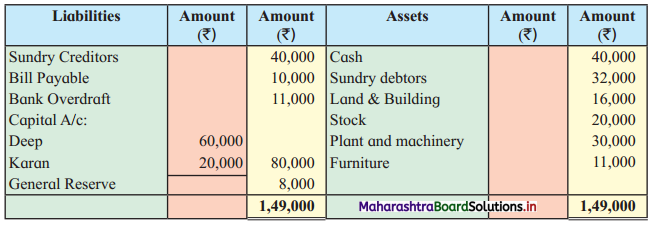They admit Shubham into Partnership on 1 April 2018. The terms being that:
1. He shall have to bring in ₹ 20,000 as his capital for 1/5 share in future profits & ₹ 10,000 as his share of Goodwill.
2. A provision for 5% doubtful debts to be created on sundry debtors.
3. Furniture to be depreciated by 20%.
4. Stock should be appreciated by 5% and Building be appreciated by 20%.
5. Capital A/c of all partners be adjusted in their new profit sharing ratio through cash account.
Prepare Profit and Loss-Adjustment A/c, Partners’ Capital A/c, Balance Sheet of the new firm.
Solution:
In the books of the firm __________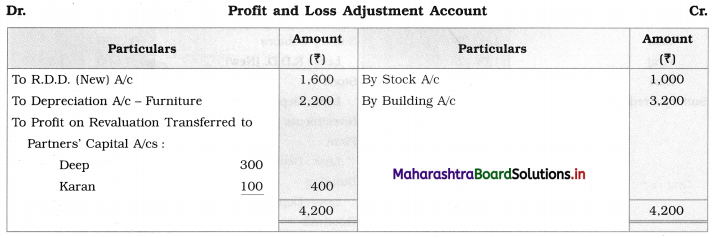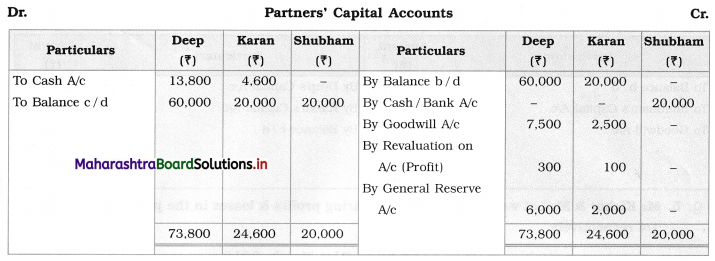Balance Sheet as of 1st April 2018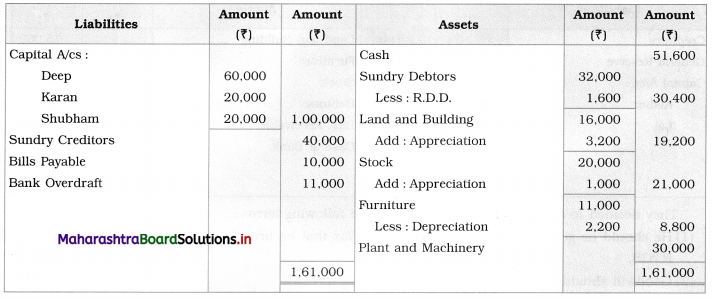Working Note:
Calculation of new ratio : Balance of 1 = 1 – share of new partner
= 1 – $$\frac{1}{5}$$
= $$\frac{4}{5}$$ (Remaining share)
New ratio = Old ratio × balance 1 (Remaining share)
Deep’s new ratio = $$\frac{3}{4} \times \frac{4}{5}=\frac{3}{5}$$
Karan’s new ratio = $$\frac{1}{4} \times \frac{4}{5}=\frac{1}{5}$$
Shubham’s new ratio = $$\frac{1}{5}=\frac{1}{5}$$
∴ New profit and loss sharing ratio = 3 : 1 : 1
Capital amount to be adjusted in Partner’s new profit and loss ratio:
Total Capital of the firm = (Reciprocal of New partner’s share) × (New partner’s capital)
= 5 × 20,000
= ₹ 1,00,000
Deep’s capital balance = $$\frac {3}{5}$$ × 1,00,000 = ₹ 60,000
Karan’s capital balance = $$\frac {1}{5}$$ × 1,00,000 = ₹ 20,000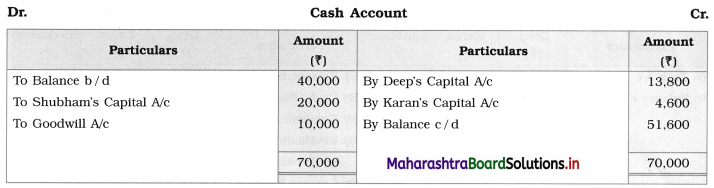Question 5.
Mr. Kishor & Mr. Lai were in partnership sharing profits & losses in the proportion of 3/4 and 1/4 respectively.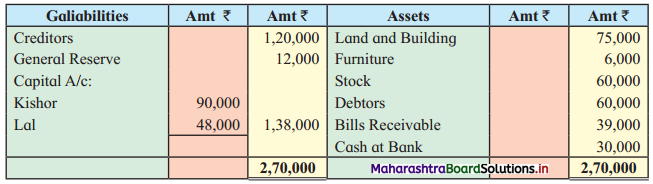They decided to admit Ram on 1 April 2018 on the following terms:
1. He should be given 1/5th share in profit and for that, he brought in ₹ 60,000 as capital through RTGS.
2. Goodwill should be raised at ₹ 60,000.
3. Appreciate Land and Building by 20%.
4. Furniture and Stock are to be depreciated by 10%.
5. The capitals of all partners should be adjusted in their new profit sharing ratio through Bank A/c.
Pass necessary Journal Entries in the books of the partnership firm and a Balance Sheet of the new firm.
Solution:
Journal entries in the books of the firm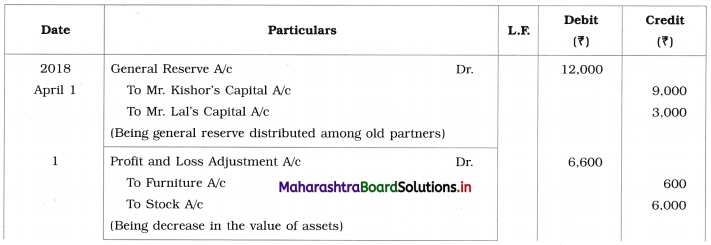Balance Sheet as of 1st April 2018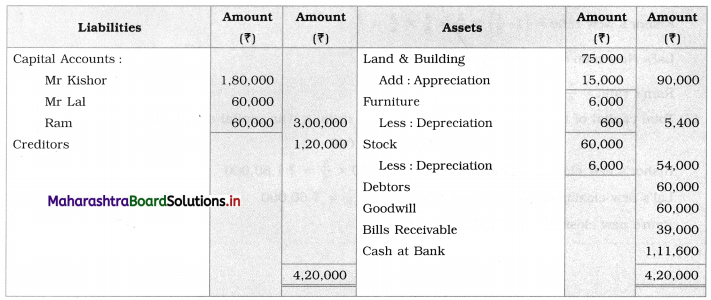Working Notes:
1.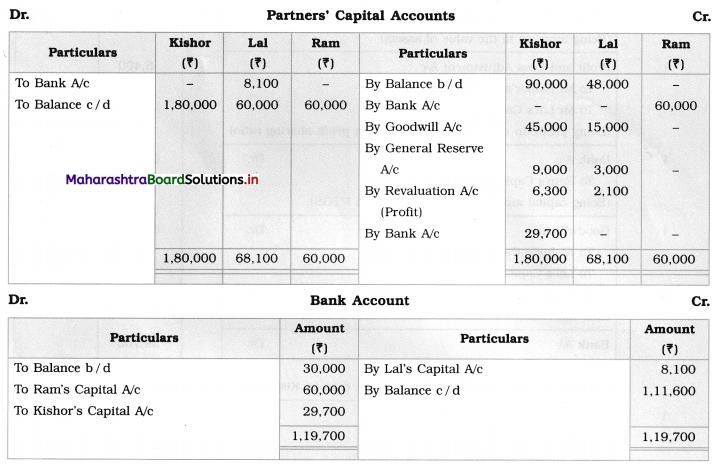2. Calculation of new profit sharing ratio:
New Ratio = (Balance of 1) × (old ratio)
Kishor’s New ratio = $$\left(1-\frac{1}{5}\right) \times \frac{3}{4}=\frac{4}{5} \times \frac{3}{4}=\frac{3}{5}$$
Lal’s New ratio = $$\left(1-\frac{1}{5}\right) \times \frac{1}{4}=\frac{4}{5} \times \frac{1}{4}=\frac{1}{5}$$
Ram’s ratio = $$\frac{1}{5}$$

3. Total capital of the firm = (Reciprocal of Ram’s ratio) × (His capital contribution)
= $$\frac{5}{1}$$ × 60,000 = ₹ 3,00,000
Kishor’s new closing capital balance = 3,00,000 × $$\frac{3}{5}$$ = ₹ 1,80,000
Lai’s new closing capital balance = 3,00,000 × $$\frac{1}{5}$$ = ₹ 60,000
Ram’s new closing capital balance = ₹ 60,000Question 6.
Vrushali and Leena are equal partners in the business. Their Balance Sheet as of 31st March 2013 stood as under.
Balance Sheet as of 31st March 2018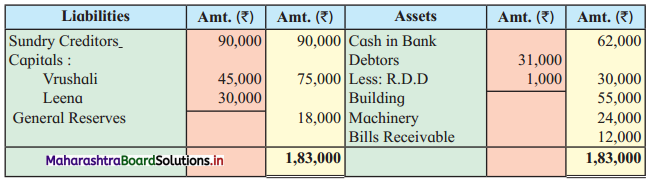They decided to admit Aparna on 1st April 2018 on the following terms:
1. The Machinery and Building be depreciated by 10%. Reserve for Doubtful Debts to be increased by ₹ 5,000.
2. Bills receivable are taken over by Vrushali at a discount of 10%.
3. Aparna should bring ₹ 60,000 as capital for her 1/4th share in future profits.
4. The Capital accounts of all the partners be adjusted in proportion to the new profit sharing ratio by opening the Current accounts of the partners.
Prepare Profit and Loss-Adjustment A/c, Partners’ Capital A/c, Balance Sheet of the new firm.
Solution: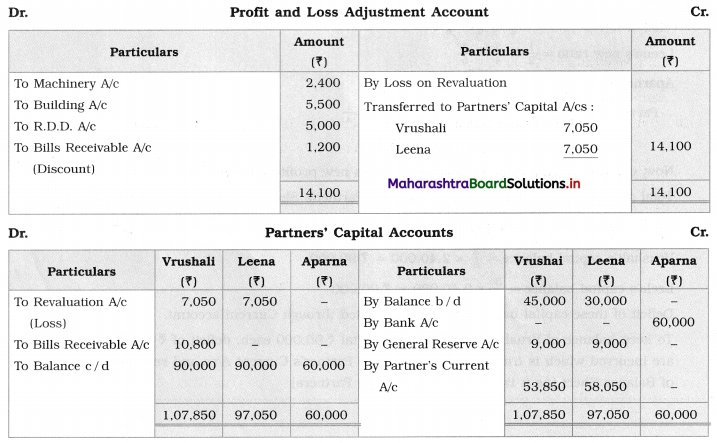Balance Sheet as on 1st April 2018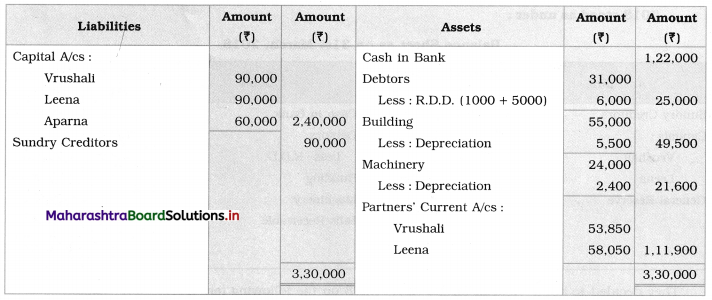Working Notes:
1. R.D.D. to be increased by ₹ 5,000 means subtract ₹ 5,000 from Debtors.

2. Bills receivable taken by Vrushali at 10 % discount i.e. 12,000 – 1,200 = ₹ 10,800.
Write this amount on the debit side of the partners’ Capital Account in Vrushali’s column.

3. Calculation of new ratio = 1 – share of new partner
= 1 – $$\frac{1}{4}$$
= $$\frac{3}{4}$$ (Remaining share)
New ratio = Old ratio × Balance 1 (Remaining Share)
Vrushali’s new ratio = $$\frac{1}{2} \times \frac{3}{4}=\frac{3}{8}$$
Leena’s new ratio = $$\frac{1}{2} \times \frac{3}{4}=\frac{3}{8}$$
Aparna’s ratio = $$\frac{1}{4}$$
∴ Partner’s new profit and loss ratio = $$\frac{3}{8}: \frac{3}{8}: \frac{1}{4}$$ = 3 : 3 : 2
Now, capital amount to be adjusted in partners new profit and loss ratio.
Total capital of the firm = (Reciprocal of New Partner’s Share) × (New Partner’s Capital)
= (Reciprocal of $$\frac{1}{4}$$) × 60,000
= 4 × 60,000
= ₹ 2,40,000
Vrushali’s capital balance = $$\frac {3}{8}$$ × 2,40,000 = ₹ 90,000
Leela’s capital balance = $$\frac {3}{8}$$ × 2,40,000 = ₹ 90,000
The deficit of these capital balances is to be adjusted through the Current account.
To keep the balance of Vrushali’s and Leena’s capital ₹ 90,000 each, deficit of ₹ 53,850 and ₹ 58,050 are incurred which is transferred to the respective Partner’s Current A/cs and recorded on the Asset side of Balance Sheet [As it is to be recovered from Partners].

Question 7.
The balance sheet of Medha and Radha who share profit and loss in the ratio 3 : 1 is as follows:
Balance Sheet as of 31st March 2018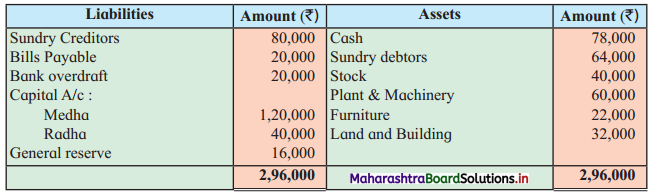They decided to admit Krutika on 1st April 2018 on the following terms:
1. Krutika is taken as a partner on 1st April 2017. She will pay 40,000 as her capital for 1/5th share in future profits and ₹ 2,500 as goodwill.
2. 5% provision for bad and doubtful debt be created on debtors.
3. Furniture be depreciated by 20%.
4. Stocks be appreciated by 5% and plant & machinery by 20 %.
5. The Capital accounts of all partners be adjusted in their new profit sharing ratio by adjusting the amount through a loan.
6. The new profit sharing ratio will be 3/5 : 1/5 : 1/5 respectively.
You are required to prepare Profit and Loss-Adjustment A/c, Partners’ Capital A/c, Balance Sheet of the new firm.
Solution: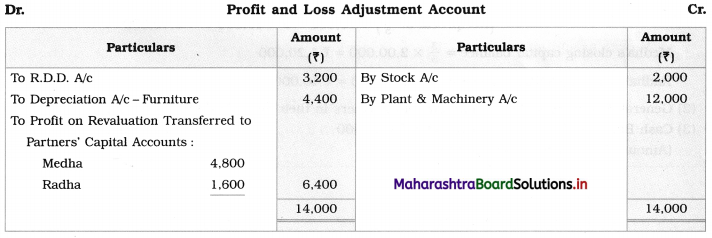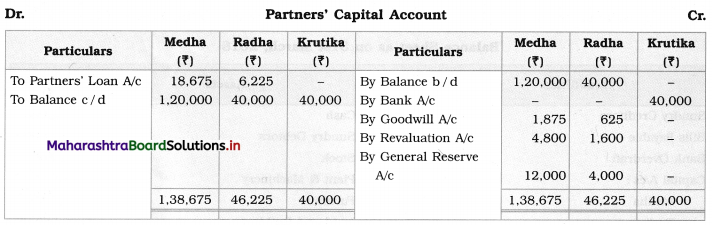Balance Sheet as of 1st April 2018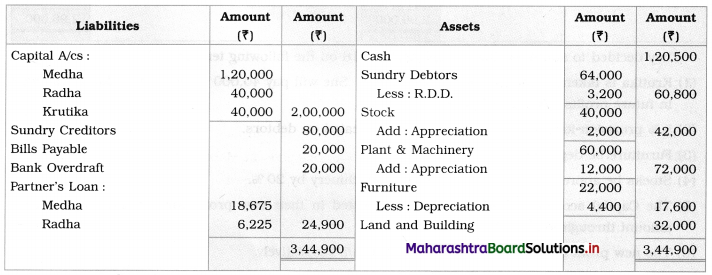Working Notes:
1. Total capital of the firm = (Reciprocal of New Partner’s Profit Sharing ratio) × (Capital contributed by new partner)
= (Reciprocal of $$\frac{1}{5}$$) × 40,000
= 5 × 40,000
= ₹ 2,00,000
Medha’s closing capital, balance = $$\frac{3}{5}$$ × 2,00,000 = ₹ 1,20,000
Radha’s closing capital balance = $$\frac{1}{5}$$ × 2,00,000 = ₹ 40,000

2. General reserve is distributed among old partners in their old profit and loss ratio.

3. Cash Balance = 78,000 + 40,000 + 2,500 = ₹ 1,20,500 [Amount brought in by new partner.]Question 8.
The Balance Sheet of Sahil and Nikhil who share profits in the ratio of 3 : 2 as of 31st March 2017 is as follows:
Balance Sheet as of 31st March 20171. Varad was to pay ₹ 1,00,000 for his share of capital.
2. He was also to pay ₹ 40,000 as his share of goodwill.
3. The new profit sharing ratio was 3 : 2 : 3.
4. Old partners decided to revalue the assets as follows:
Building ₹ 1,00,000. Furniture ₹ 48,000, Debtors ₹ 38,000 (in view of likely bad debts)
5. It was found that there was a liability for ₹ 3,000 for goods in March 2017 but recorded on 2nd April 2017.
You are required to prepare:
(b) Capital accounts of the partners
Solution: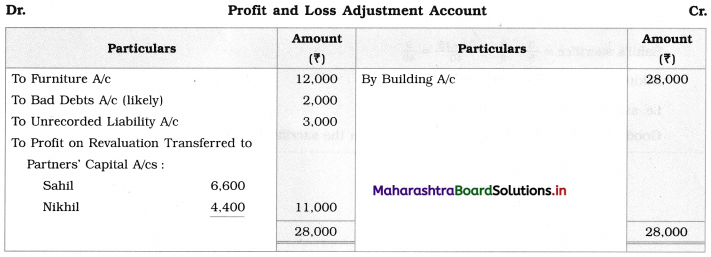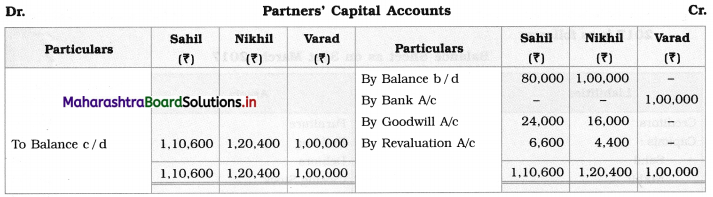Balance Sheet as of 1st April 2017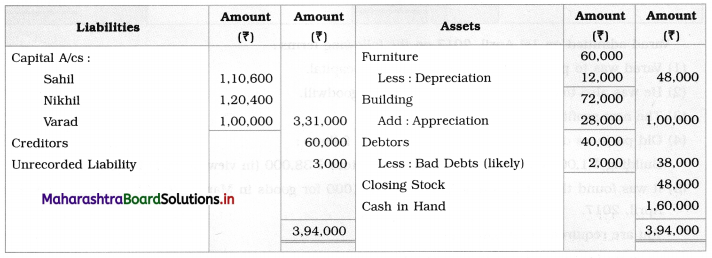Working Notes:
1. Cash in hand = Opening balance + Varad’s capital + Varad’s goodwill (amount brought in)
= 20,000 + 1,00,000 + 40,000
= ₹ 1,60,000

2. Sacrifice ratio = Old ratio – New ratio
Sahil’s sacrifice = $$\frac{3}{5}-\frac{3}{8}=\frac{24-15}{40}=\frac{9}{40}$$
Nikhil’s sacrifice = $$\frac{2}{5}-\frac{2}{8}=\frac{16-10}{40}=\frac{6}{40}$$
i.e. sacrifice ratio = $$\frac{9}{40}: \frac{6}{40}$$ = 9 : 6 = 3 : 2.
Goodwill is distributed among old partners in the sacrifice ratio.

Question 9.
Mr. Amit and Baban share profits and losses in the ratio 2 : 3 respectively. Their Balance Sheet as of 31st March 2018 was as under:
Balance Sheet as of 31st March 2018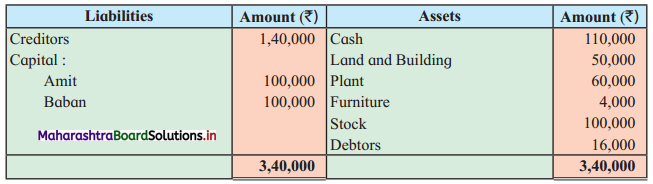They agreed decided to admit Kamal on 1st April 2018 on the following terms:
1. Kamal shall have 1/4th share in future profits.
2. She shall bring 50,000 as her capital and 40,000 as her share of goodwill.
3. Land and building to be valued at 60,000 and furniture to be depreciated by 10%.
4. Provision for bad and doubtful debts is to be maintained at 5% on the sundry debtors.
5. Stocks to be valued at 1,10,000.
The Capital A/c of all partners to be adjusted in their new profit and loss ratio and excess amount be transferred to their loan accounts.
Prepare Profit and Loss-Adjustment A/c, Capital A/cs, and New Balance Sheet.
Solution: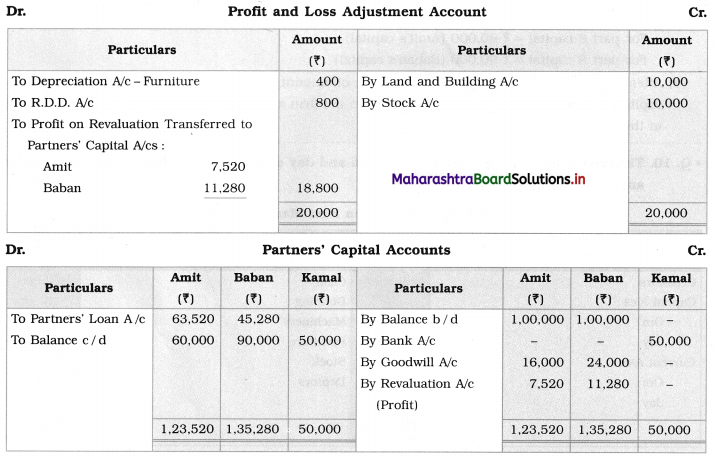Balance Sheet as of 1st April 2018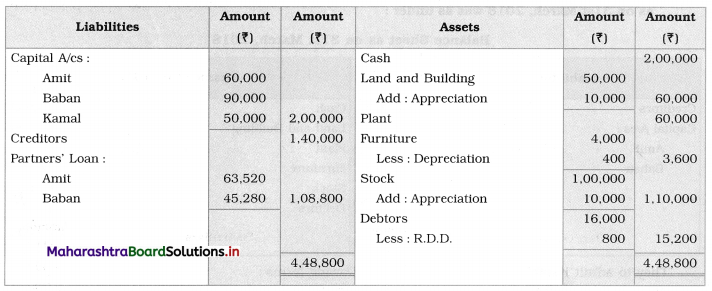Working Notes:
1. Cash balance = Opening balance + Amount brought in by Kamal
= 1,10,000 + 50,000 + 40,000
= ₹ 2,00,000

2. For calculation of new profit and loss ratio:
Calculation of new profit ratio = 1 – share of new partner
= 1 – $$\frac{1}{4}$$
= $$\frac{3}{4}$$ (Remaining share)
New ratio = old ratio × balance 1 (Remaining share)
Amit’s new ratio = $$\frac{2}{5} \times \frac{3}{4}=\frac{6}{20}$$
Baban’s new ratio = $$\frac{3}{5} \times \frac{3}{4}=\frac{9}{20}$$
Kamal’s ratio = $$\frac{1}{4}=\frac{1}{4} \times \frac{5}{5}=\frac{5}{20}$$

3. New profit and loss ratio = 6 : 9 : 5
Capital amount adjusted in their new profit and loss ratio by taking new partner Kamal’s capital (₹ 50,000) as a base.
For part 5 capital = ₹ 50,000 (Kamal’s capital)
For part 6 capital = ₹ 60,000 (Amit’s capital)
For part 9 capital = ₹ 90,000 (Baban’s capital)

4. After keeping these capital balances difference of the amount of Amit’s capital ₹ 63,520 and of Baban’s capital ₹ 45,280 are taken as partner’s loan to the firm and as a liability of the firm it is recorded in the Liabilities side of the Balance Sheet.Question 10.
The following is the Balance Sheet of Om and Jay on 31st March 2018, they share profits and losses in the ratio 3 : 2.
Balance Sheet as of 31st March 2018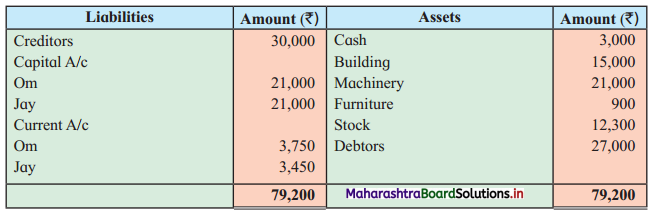They take Jagdish into partnership on 1st April 2018. The terms being:
1. Jagdish should pay ₹ 3,000 as his share of Goodwill. 50% of goodwill withdrawn by partners in cash.
2. He should bring ₹ 9,000 as capital for 1/4th share in future profits.
3. Building to be valued at 18,000, Machinery and Furniture to be reduced by 10 %.
4. A provision of 5% on debtors to be made for doubtful debts.
5. Stock to be taken at the value of ₹ 15,000.
Prepare Profit and Loss A/c, Partners’ Current A/c, Balance Sheet of the new firm.
Solution: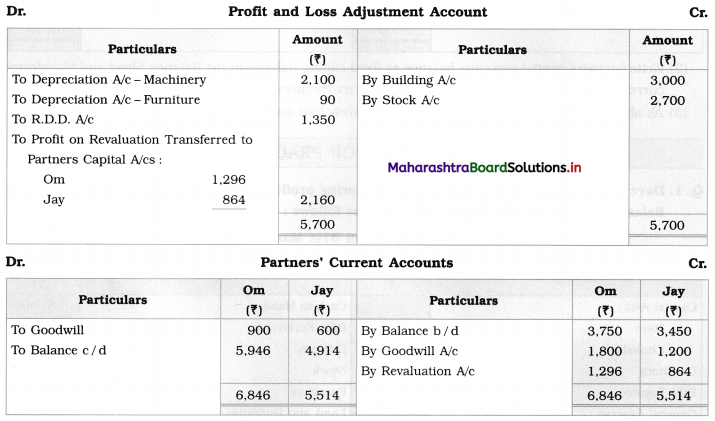Balance Sheet as of 1st April 2018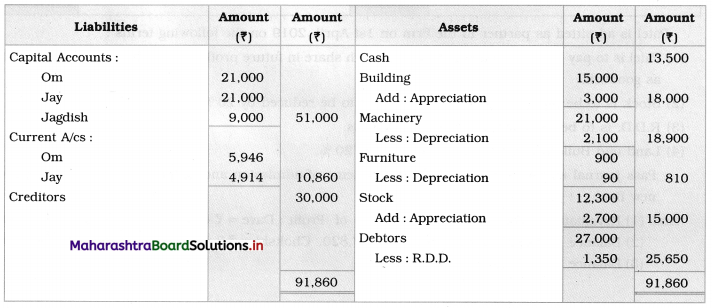Working Notes:
1.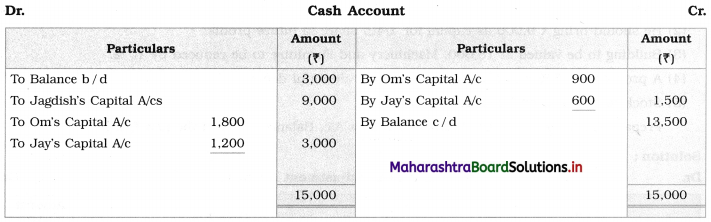2. Write partner’s capital accounts balance as fixed capital balance in the Balance Sheet and transferred current account balance in the Balance Sheet as Partners Current A/c.

3. As shown in the cash account partners’ withdrew half amount of goodwill amount share.